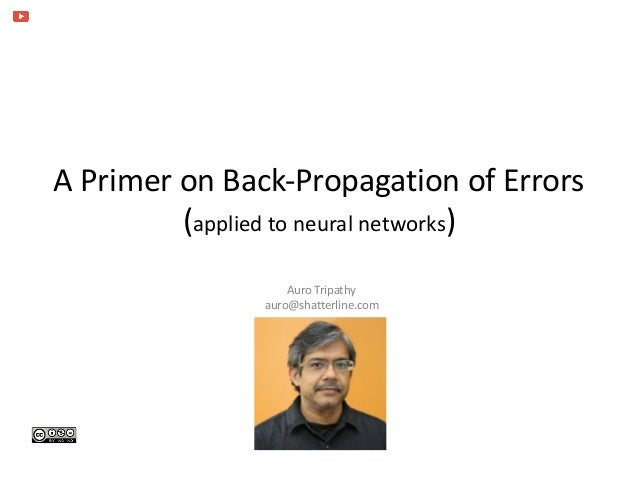Successfully reported this slideshow.Upcoming SlideShare
×

# Back-propagation Primer

809 views

Published on

Welcome to a primer on the back-propagation (of errors) as it applies to the training of neural networks. We answer the question, what's the contribution of the back-propagation-technique?

Published in: Education
• Full Name
Comment goes here.

Are you sure you want to Yes NoAre you sure you want to  Yes  No

### Back-propagation Primer

1. 1. A Primer on Back-Propagation of Errors  (applied to neural networks) Auro Tripathy auro@shatterline.com
2. 2. Auro Tripathy Outline • Summary of Forward-Propagation • The Calculus of Back-propagation • Summary 2
3. 3. Auro Tripathy A Feed-Forward Network is a Brain-Inspired Metaphor 3
4. 4. Auro Tripathy Feed-forward to calculate the error relative to the desired output  Error-Function (aka Loss-, Cost-, or Objective-Function) • In the feed-forward path, calculate the error relative to the desired output • We define a error-function E(X3, Y) as the “penalty” of predicting X3 when the true output is Y. • The objective is to minimize the error across all the training samples. • The error/loss E(X3, Y) assigns a numerical score (a scalar) for the network’s output X3 given the expected output Y. • The loss is zero only for cases where the neural network’s output is correct. 4
5. 5. Auro Tripathy Sigmoid Activation Function The sigmoid activation function σ(x) = 1/(1 + e−x) is an S-shaped activation function transforming all values of x in the range, [0,1] 5 https://en.wikipedia.org/wiki/File:Logistic-curve.svg
6. 6. Auro Tripathy Gradient Descent 6 Note, in practice, we don’t expect a global minima, as shown here a b
7. 7. Auro Tripathy “Unshackled by the chain-rule”  -Patrick Winston, MIT 7
8. 8. Auro Tripathy Derivative of the Error E with-respect-to the Output, X3 8
9. 9. Auro Tripathy Derivative of the Sigmoid Activation Function 9 P3 X3 For the Sigmoid function, the cool thing is, the derivative of the output, X3 (with respect to the input, P3) is expressed in terms of the output, i.e., X3 . (1 - X3) http://kawahara.ca/wp-content/uploads/derivative_of_sigmoid.jpg
10. 10. Auro Tripathy Derivative of P3 with-respect-to W3 10
11. 11. Auro Tripathy Propagate the errors backward and adjust the weights, w, so the actual output mimics the desired output 11
12. 12. Auro Tripathy Computations are Localized & Partially Pre-computed in the Previous Layer 12
13. 13. Auro Tripathy Summary ☑If there’s a representative set of inputs and outputs, then back-propagation can learn the the weights. ☑Back-propagation has linear performance relative to the number of layers. ☑Simple to implement (and test) 13
14. 14. Auro Tripathy Credits 14 Concepts crystalized from MIT Professor Patrick Winston’s lecture, https://www.youtube.com/watch?v=q0pm3BrIUFo auro@shatterline.com